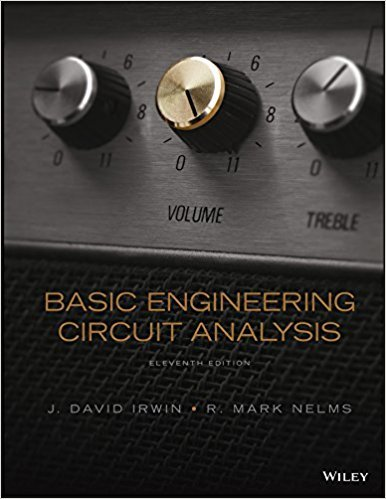×
Get Full Access to Basic Engineering Circuit Analysis - 11 Edition - Chapter 6 - Problem 6.7
Get Full Access to Basic Engineering Circuit Analysis - 11 Edition - Chapter 6 - Problem 6.7

×

# Find the total inductance at the terminals of the networkISBN: 9781118539293 159

## Solution for problem 6.7 Chapter 6

Basic Engineering Circuit Analysis | 11th Edition

• Textbook Solutions
• 2901 Step-by-step solutions solved by professors and subject experts
• Get 24/7 help from StudySoup virtual teaching assistantsBasic Engineering Circuit Analysis | 11th Edition

4 5 1 304 Reviews
28
3
Problem 6.7

Find the total inductance at the terminals of the network in Fig. P6.70. 12 mH 6 mH 4 mH 2 mH LT 12 mH Figure P6.70

Step-by-Step Solution:
Step 1 of 3

Three Cases I: Simple Real Roots n N(s) A j I: Simple Real Roots F s n    ss j j1ss j II: Repeated Real Roots j1 III: Complex Conjugate and Simple Real Roots Aiss)F(si ssi 1

Step 2 of 3

Step 3 of 3

##### ISBN: 9781118539293

The full step-by-step solution to problem: 6.7 from chapter: 6 was answered by , our top Engineering and Tech solution expert on 11/23/17, 05:00AM. Basic Engineering Circuit Analysis was written by and is associated to the ISBN: 9781118539293. Since the solution to 6.7 from 6 chapter was answered, more than 246 students have viewed the full step-by-step answer. The answer to “Find the total inductance at the terminals of the network in Fig. P6.70. 12 mH 6 mH 4 mH 2 mH LT 12 mH Figure P6.70” is broken down into a number of easy to follow steps, and 26 words. This textbook survival guide was created for the textbook: Basic Engineering Circuit Analysis, edition: 11. This full solution covers the following key subjects: fig, figure, Find, inductance, Network. This expansive textbook survival guide covers 15 chapters, and 1430 solutions.

Unlock Textbook Solution